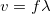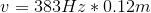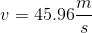# High School Physics : Understanding Calculations with Velocity

## Example Questions

### Example Question #1 : Understanding Calculations With Velocity

A spring oscillates back and forth with a period of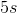. If its wavelength is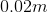, what is the speed of the spring?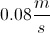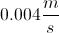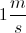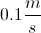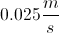Explanation:

Velocity is commonly described with respect to wavelength and frequency.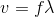We are given the period and the wavelength. Period is simply the inverse of freqeuncy.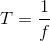Using this, we can rewrite our original equation in terms of the period.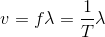Now we can use the values given in the question to solve for the velocity.

Plug in our given values and solve.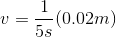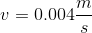### Example Question #2 : Understanding Calculations With Velocity

A wave oscillates with a frequency of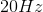. If it has a speed of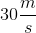, what is its wavelength?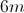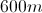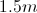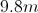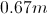Explanation:

The equation for velocity in terms of wavelength and frequency is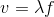.

We are given the velocity and frequency. Using these values, we can solve for the wavelength.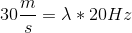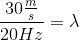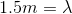### Example Question #41 : Waves

A note is played in a gas (which is not a normal atmosphere). Inside of this gas, the note has a frequency of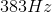and a wavelength of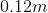. What is the speed of sound in this gas?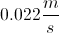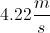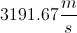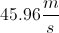Explanation:

The relationship between velocity, frequency, and wavelength is:Plug in the given information to solve: